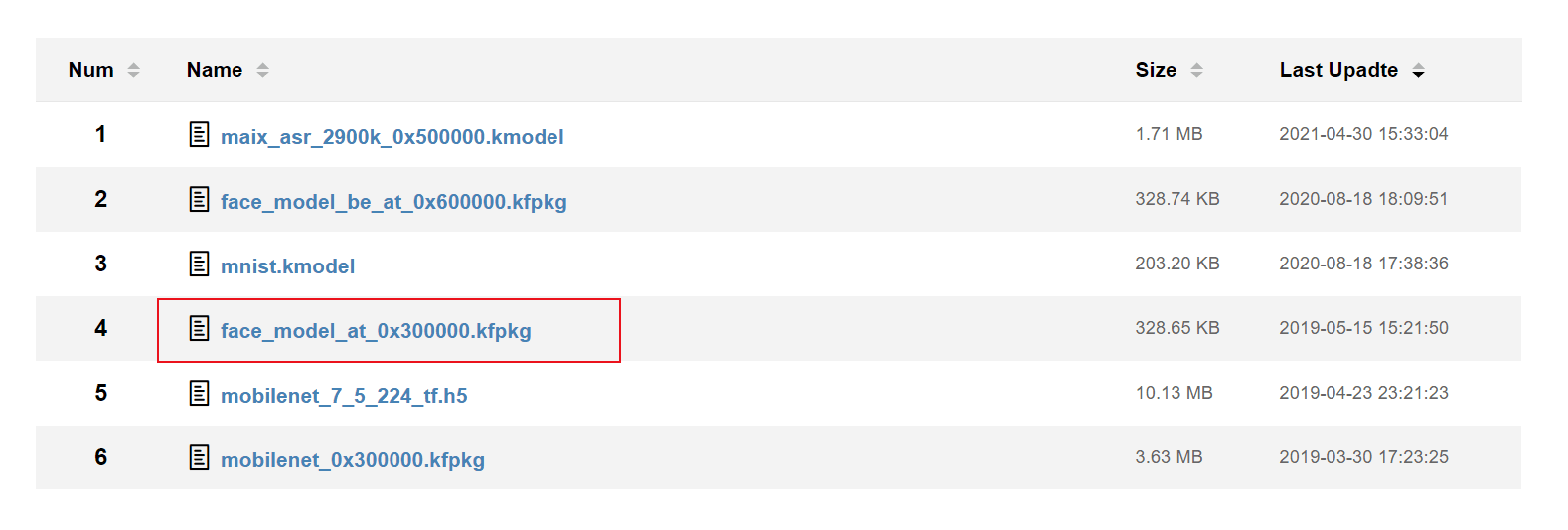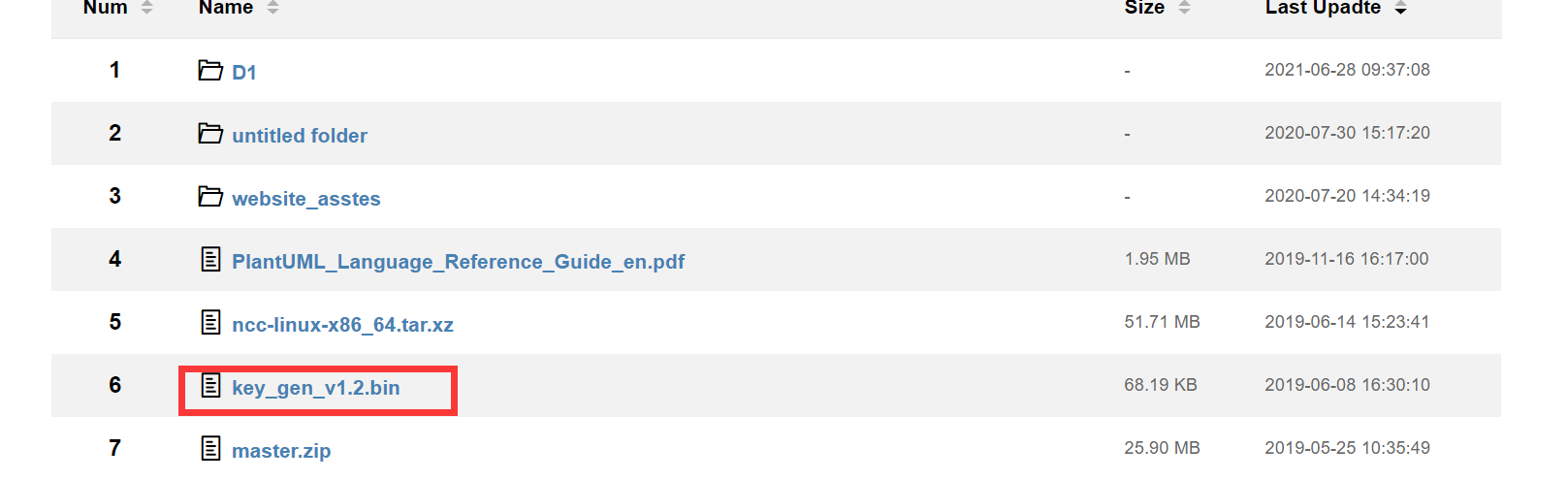# 一、KPU模块方法

1、

``````KPU.load(offset, file_path)
``````

offset: 模型在 flash 中的偏移大小，如 0xd00000 表示模型烧录在13M起始的地方, 0x300000表示在 Flash 3M的地方。
file_path: 模型在文件系统中为文件名， 如 “/sd/xxx.kmodel”

kpu_net: kpu 网络对象

2、

``````kpu.load_flash(model_addr, is_dual_buf, batch_size, spi_speed)
``````

is_dual_buf：0,单一缓冲区加载，使用较少的 RAM 和较慢的速度动态加载该模型文件； 1，开启双缓冲加载，需要更大的 RAM， 运行速度相对较快。
batch_size：将 is_dual_buf 设置为 1 时，需要设置 load batch_size，建议值为 0x4000~0x10000，可以测试出模型的最佳值。如果 is_dual_buf 为 0 则设置为 0
spi_speed：使用 SPI flash 加载模型文件时，我们会暂时将 flash 设置为高速模式，并设置所需的 spi 时钟频率。该值应 <= 80000000(实际频率，设值可能不等于实际频率。)

kpu_net: kpu 网络对象

3、

``````KPU.init_yolo2(kpu_net, threshold, nms_value, anchor_num, anchor)
``````

``````import KPU as kpu
anchor = (1.889, 2.5245, 2.9465, 3.94056, 3.99987, 5.3658, 5.155437, 6.92275, 6.718375, 9.01025)
``````

threshold: 概率阈值， 只有是这个物体的概率大于这个值才会输出结果， 取值范围：[0, 1]
nms_value: box_iou 门限, 为了防止同一个物体被框出多个框，当在同一个物体上框出了两个框，这两个框的交叉区域占两个框总占用面积的比例 如果小于这个值时， 就取其中概率最大的一个框
anchor_num: anchor 的锚点数， 这里固定为 len(anchors)//2
anchor: 锚点参数与模型参数一致，同一个模型这个参数是固定的，和模型绑定的（训练模型时即确定了）， 不能改成其它值。

success： bool类型， 是否成功

4、

``````KPU.deinit(kpu_net)
``````

``````import KPU as kpu
import gc
gc.collect()
``````

``````import KPU as kpu
import gc
gc.collect()
``````

success： bool 类型， 是否成功

5、

``````kpu.run_yolo2(task, img)
``````

``````import KPU as kpu
import image
anchor = (1.889, 2.5245, 2.9465, 3.94056, 3.99987, 5.3658, 5.155437, 6.92275, 6.718375, 9.01025)
img = image.Image()
``````

image_t：从 sensor 采集到的图像

list: kpu_yolo2_find 的列表

# 二、人脸检测``````sensor.set_vflip(sensor_vflip)
#修改为
sensor.set_vflip(1)
``````

MAIX BIT(K210)人脸检测

# 二、人脸识别

## 1.获取机器码

， 获得模型smodel, 就是 加密版本的kmodel。下载后缀为.smodel模型需要用到机器码。机器码是一机一码的一种加密方式，用于模型文件的加密。如果使用别的机器码去加密或者下载以 smodel 为文件后缀的模型文件，开发板是无法使用该模型文件的。

1、将 key_gen.bin 这个固件通过 Kflash 烧录到开发板上。烧录这个机器码固件之后，开发板是处于一个不能使用的状态（就是什么功能都不能使用，包括IDE），上电屏幕只会变成一个白屏。2、

3、

k210机器码获取

## 2.使用方法

``````if code:
for i in code:
# Cut face and resize to 128x128
a = img.draw_rectangle(i.rect())
face_cut = img.cut(i.x(), i.y(), i.w(), i.h())
face_cut_128 = face_cut.resize(128, 128)
a = face_cut_128.pix_to_ai()
# a = img.draw_image(face_cut_128, (0,0))
# Landmark for face 5 points
plist = fmap[:]
le = (i.x() + int(plist * i.w() - 10), i.y() + int(plist * i.h()))
re = (i.x() + int(plist * i.w()), i.y() + int(plist * i.h()))
nose = (i.x() + int(plist * i.w()), i.y() + int(plist * i.h()))
lm = (i.x() + int(plist * i.w()), i.y() + int(plist * i.h()))
rm = (i.x() + int(plist * i.w()), i.y() + int(plist * i.h()))
a = img.draw_circle(le, le, 4)
a = img.draw_circle(re, re, 4)
a = img.draw_circle(nose, nose, 4)
a = img.draw_circle(lm, lm, 4)
a = img.draw_circle(rm, rm, 4)
# align face to standard position
src_point = [le, re, nose, lm, rm]
T = image.get_affine_transform(src_point, dst_point)
a = image.warp_affine_ai(img, img_face, T)
a = img_face.ai_to_pix()
# a = img.draw_image(img_face, (128,0))
``````

1、检测到人脸
2、裁出人脸，找到人脸的眼睛鼻子嘴巴， 这里裁成了128×128的图
3、把人脸图中的脸旋转到标准位置
4、用特征提取模型提取出人脸的特征值

maix bit人脸识别

# 总结

MAIX BIT（K210）上可以进行人脸检测和人脸识别，相比来说，人脸检测更加容易实现，人脸识别虽然能识别出人脸，但是识别出是同一人的概率较低，后期可以更换模型来达到更好的效果。